## pdsolve exception (in GAMMA) numeric exception: di...

This is new exception generated by Maple pdsolve in 2021. Different from the last post I gave on pdsolve. So I thought it will be better to keep them separate since the causes are different.

```interface(version)
restart;
pde :=  diff(w(x,y,z),x)+(a1*x^n1*y+b1*x^m1)*diff(w(x,y,z),y)+(a2*x^n2*y+b2*x^m1)*diff(w(x,y,z),z)= 0;
pdsolve(pde,w(x,y,z));

#another example

restart;
local gamma:
pde := diff(w(x,y,z),x)+(a1*x^n1*y+ b1*x^m1)*diff(w(x,y,z),y)+(a2*x^n2*y+b2*x^m2)*diff(w(x,y,z),z)=c2*x^k2*y+c1*x^k1*z;
pdsolve(pde,w(x,y,z));

```

Error, (in GAMMA) numeric exception: division by zero

The same PDE works in 2020.2. The answer it gives is large so will not show it all below.

Screen shots

Maple 2021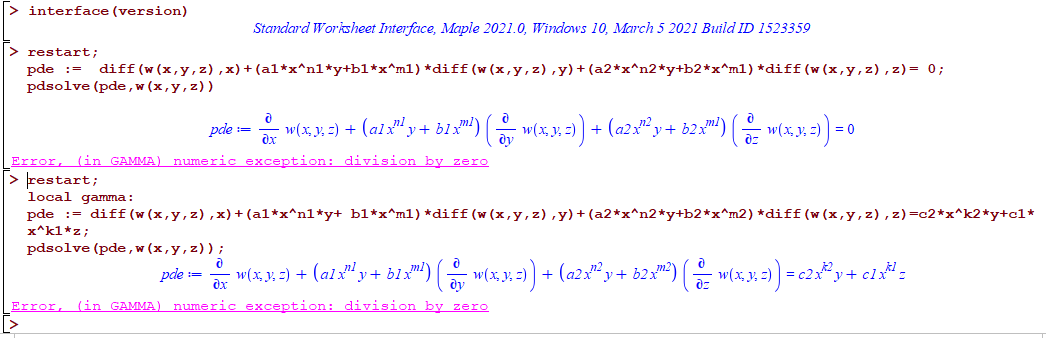Maple 2020.2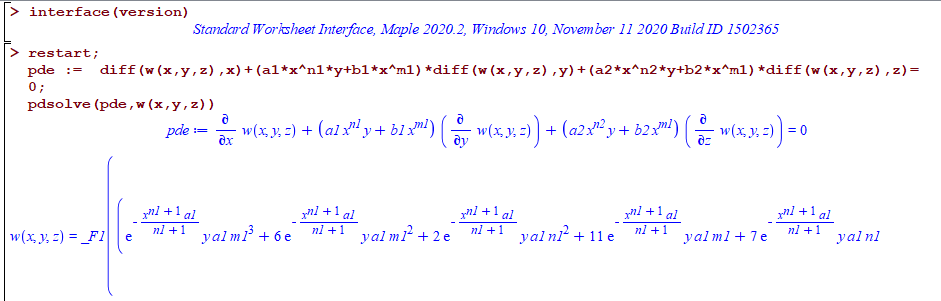All on windows 10.

Do other see the same error? What causes it?

## seq - numelems and declaring a local variable in ...## pdsolve exception int/gbinthm/structure INVALID s...

I noticed number of pde's now fail in Maple 2021 with the error

int/gbinthm/structure INVALID subscript selector

but they do not fail in Maple 2020.2.

Here are few   examples

```restart;
pde :=a*x^n*diff(w(x,y),x) + n*x^m*y*diff(w(x,y),y) =s*x^p*y^q+d;
pdsolve(pde,w(x,y));

restart;
pde :=a*x^n*diff(w(x,y),x) + n*x^m*y*diff(w(x,y),y)=c*x^k*y^s+d;
pdsolve(pde,w(x,y))

restart;
pde :=a*x^n*diff(w(x,y),x)+b*x^m*y*diff(w(x,y),y) =  (c*x^k*y^s + d)*w(x,y);
pdsolve(pde,w(x,y))

restart;
pde :=  a*diff(w(x,y),x)+ y*diff(w(x,y),y) = b*w(x,y)+ c*x^n*y^m;
pdsolve(pde,w(x,y))

#etc..```

Error, (in int/gbinthm/structure) invalid subscript selector

While in Maple 2020.2 they all work. Screen shot

Maple 2021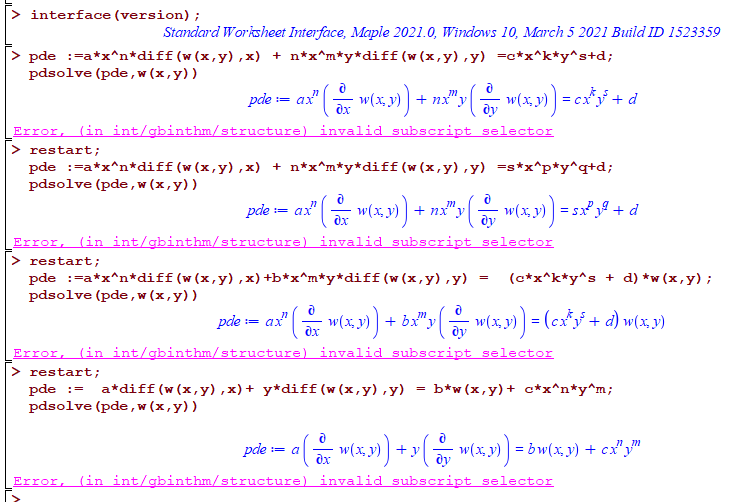Maple 2020.2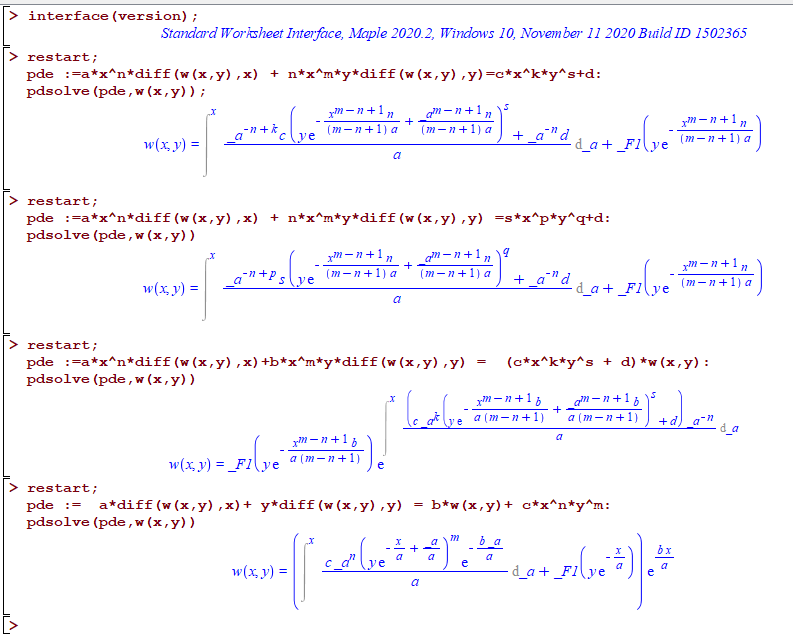Any idea why this happens? Do others see the same error?

## Maple 2021 / Problem while saving the workbook...

I've experienced problems a couple of times when trying to save a workbook in Maple 2021.

At first I thought it was due to a unstable VPN connection, but this also happens on a local drive.## why the new documentation PDF's for Maple 2021 are...

This is just an obervation. May be due to me missing some clarification.

My understanding is that in Maple 2021, one can now export a complete Maple worksheet/document to Latex successfuly.

According to https://www.maplesoft.com/products/maple/new_features/  it says under "Latex export"

Export is available for individual expressions or the entire document, though the latex command

So I would have expected that now all the online PDF documents for the new features in Maple 2021 to be generated from Latex for better formatting.

But when going over number of documents, they do not appear to have converted to Latex before generating the PDF.

Even the PDF document about the new latex command does not appear to have been generated from Latex. I can quickly recognize a PDF that was generated from Latex, and these PDF files are clearly not.

Looking at sample PDF from Maple website, it says the generator is "iText" and not latex compiler.

It looks like these documents were just a direct export of the Maple worksheet to PDF, instead of being exporting to Latex first, and then compiled to PDF.

Am I missing something? Why not take advantage of the Maple Latex export, and convert all the documents to Latex first and then generate the PDF from Latex? This would make them look much better in terms of formatting and math rendering.

Here are some examples

Why did not Maple documentation take advantage of the new export command of Maple's latex command? This would also have been a good way and an opportunity to show case the effectiveness of the new latex export command.

May be it was not done due to time constraint before the release date of maple 2021? Or are there other technical reasons?

## Bug in Integration of square of Jacobi sine functi...

Dear all,

I believe there is a bug in integration of square of Jacobi sine function.

Here is what I did:

u := int(JacobiSN(x, k)^2, x);

The result I got is
x - EllipticE(JacobiSN(x, k), k)
u := --------------------------------
k^2
However, according to  Handbook of elliptic integrals for engineers and scientists by Byrd and Friedman Eq (310.02), it is supposed to be

x - EllipticE(JacobiAM(x, k), k)
u := --------------------------------
k^2

Note that I tried also to integrate first and third power of JacobiSN a the results are in agreement with the book.

## Some bug with Physics and int in Maple 2021...

Hi there.

I found some strange behavior of int function in document with using of Physics package in Maple 2021 in comparison with Maple 2020:

kerr.mw

Please look at that.

Thank you.

## How do I Int(pde) in Maple?...

Int(UxUxy+UxxUy, x)=UxUy

How can I do this?

## Polynomials: How do I determine the linear factors...

Show that x-2 is a factor of p(x)=x^3+3x^2-4x-12

I)determine all the linear factors of p(x)

ii)what are the zeros of y=p(x)

iii)sketch function y=p(x)

## issue using simplify on result of integration in ...

In Maple 2021

```restart;
B:=sqrt( (-4*u^(1/3)+1)*u^(4/3));
A:=1/(-12*u+3*u^(2/3)-3*B);
res:=int(A,u);
simplify(res)
```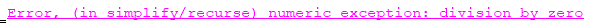This is one of the integrals, that in Maple 2020 did not handle. In Maple 2020, it gives this

```restart;
B:=sqrt( (-4*u^(1/3)+1)*u^(4/3));
A:=1/( -12*u+ 3*u^(2/3)-3*B);
res:=int(A,u);
```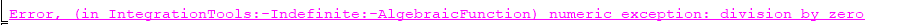The difference is that in Maple 2021 int is able to solve this (but gives very long output which I will not post here). The problem is now simplify() gives an error on the result. I was trying to see if possible to obtain the same small result as Mathematica's after simplifying. Here is Mathematica's result

```ClearAll[u];
B = Sqrt[(-4*u^(1/3) + 1)*u^(4/3)];
A = 1/(-12*u + 3*u^(2/3) - 3*B);
res = Integrate[A, u]```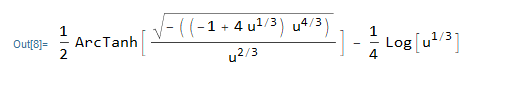It is good that Maple can solve this integral, but why simplify gives error on the result? Is there a workaround?

## How to use Physics in Maple 2021?...

I installed Maple 2021 on windows 10. And wanted to try it to see if the hangs I used to have are fixed now.

First I noticed that Physics package does not come pre-installed with Maple 2021, which is little strange. I would have expected Maple 2021 to come with latest Physics version.

Because when I did Physics:-Version() it says "`The "Physics Updates" package is not installed`"

Then I typed  Physics:-Version(latest) to install it, it gives error

Error, (in Physics:-Version) unable to determine the Physics Updates version, could you please report the problem to support@maplesoft.com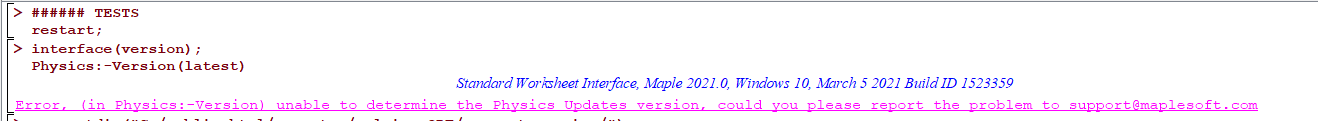I remember something similar in earlier version of Maple but can't find or remember where that post now.

May be Physics needs to be updated at Maple site to work with Maple 2021? I am asking, because Latex() does not work without Physics installed.

Edit:

I think in Maple 2021, latex() now is the same as the earlier Latex() command from Physics? I remember a post saying this now. Since I see now latex() have different help page from old latex() help page.

So may be that is why Latex() did not work. I can easily change this in my code to change it to use latex() instead of Latex() in this case.

Windows 10

## Unveiling Maple 2021

by: Maple 2021I’ll admit it. There are times when I don't fully understand every mathematical advancement each release of Maple brings. Given the breadth of what Maple does, I guess that isn't surprising.

In development meetings, I make the pretence of keeping up by looking serious, nodding knowingly and occasionally asking to go back to the previous slide “for a minute”. I’ve been doing this since 2008 and no one’s caught on yet.

But I do understand

• the joy on a user’s (Zoom) face when they finally solve a complex problem with a new version of Maple
• the smiley emojis that students send us when they understand a tricky math concept with the help of an improved Maple tutor
• and the wry smile on a developer’s face when they get to work on a project they really want to work on, and the bigger smile when that project gets positive feedback

These are all moments that give me that magic dopamine hit.

The job that Karishma and I have is to make users happy. We don’t have to be top-flight mathematicians, engineers or computer scientists to do that. We just have to know what itch to scratch.

Here’s some things I think might give you that dopamine hit when you get your hands on Maple 2021. You can also explore the new release yourself at What’s New in Maple 2021.

Worksheet mode has been my go-to interface for when I just want to get stuff done. This is mostly because worksheet mode always felt like a more structured environment for developing math when I didn’t have all the steps planned out in advance, and I found that structure helpful. I’d use Document mode when I needed to use the Context Panel for math operations and didn’t want to see the commands, or I needed to create a nice looking document without input carets. And this was fine – each mode has its own strengths and uses – but I what I really wanted was the best of both worlds in a single environment.

This year, we’ve made one change that has let me transition far more of my work into Document mode.

In Document Mode, pressing Enter in a document block (math input) now always moves the cursor to the next math input (in previous releases, the cursor may have moved to the start of the next line of text).

This means you can now quickly update parameters and see the downstream effects with just the Enter key – previously, a key benefit of worksheet mode only.

There’s another small change we’ve made - inserting new math inputs.  In previous releases of Maple, you could only insert new document blocks above the in-focus block using a menu item or a three-key shortcut.

In Maple 2021, if you move the insertion point to the left of a document block (Home position), the cursor is now bold, as illustrated here:Now, if you press Enter, the in-focus prompt is moved down and a new empty math input is created.

Once you get used to this change, Ctrl+Shift+K seems like a distance memory!

@Scot Gould logged a request that Maple numerically solve a group of differential equations collected together in a vector. And now you can!Before Maple 2021, this expression was unchanged after evaluation. Now, it is satisfyingly simpler.We’ve dramatically increased the scope of the signal processing package.

My favorite addition is the MUSIC function. With some careful tuning, you can generate a pseudo power spectrum at frequencies smaller than one sample.

First generate a noisy data set with three frequencies (two frequencies are closer than one DFT bin).

```with(SignalProcessing):
num_points:= 2^8:
sample_rate := 100.0:
T := Vector( num_points, k -> 2 * Pi * (k-1) / sample_rate, 'datatype' = 'float' ):
noisy_signal:=Vector( num_points, k -> 5 * sin( 10.25 * T[k] ) + 3 * sin( 10.40 * T[k] ) - 7 * sin( 20.35 * T[k] )) + LinearAlgebra:-RandomVector(num_points, generator=-10..10):
dataplot(noisy_signal, size = [ 800, 400 ], style = line)```Now generate a standard periodogram

`Periodogram( noisy_signal, samplerate = sample_rate, size = [800, 400] )`This approach can’t discriminate between the two closely spaced frequencies.

And now the MUSIC pseudo spectrum

`MUSIC( noisy_signal, samplerate = sample_rate, dimension = 6, output = plot );`The Maple Quantum Chemistry Toolbox from RDMChem, a separate add-on product to Maple, is a powerful environment for the computation and visualization of the electronic structure of molecules. I don’t pretend to understand most of what it does (more knowing nods are required). But I did get a kick out of its new molecular dictionary. Did you know that caffeine binds to adenosine receptors in the central nervous system (CNS), which inhibits adenosine binding? Want to know more about the antiviral drug remdesivir? Apparently it looks like this:We put a lot of work into resources for students and educators in this release, including incorporating study guides for Calculus, Precalculus, and Multivariate Calculus, a new student package for ODEs, and the ability to obtain step-by-step solutions to even more problems.  But my favourite thing out of all this work is the new SolvePractice command in the Grading Tools package.  Because it lets you build an application that does this:I like this for three main reasons:

1. It lets students practise solving equations in a way that actually helps them figure out what they’ve done wrong, saving them from a spiral of frustration and despair
2. The same application can be shared via Maple Learn for students to use in that environment if they don’t have Maple
3. The work we did to create that “new math entry box” can also be used to create other Maple applications with unknown numbers of inputs (see DocumentTools). I’m definitely planning on using this feature in my own applications.

Okay, yes, we know. Up until recently, our LaTeX export has been sadly lacking. It definitely got better last year, but we knew it still wasn’t good enough. This year, it’s good. It’s easy. It works.  And it’s not just me saying this. The feedback we got during the beta period on this feature was overwhelmingly positive.That’s just the tip of the Maple 2021 iceberg of course. You can find out more at What’s New in Maple 2021.  Enjoy!

 First26 27 28 Page 28 of 28
﻿